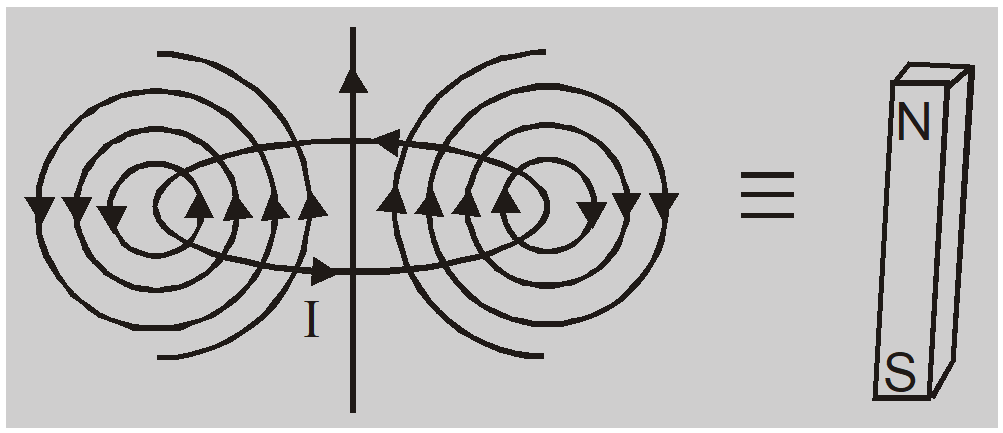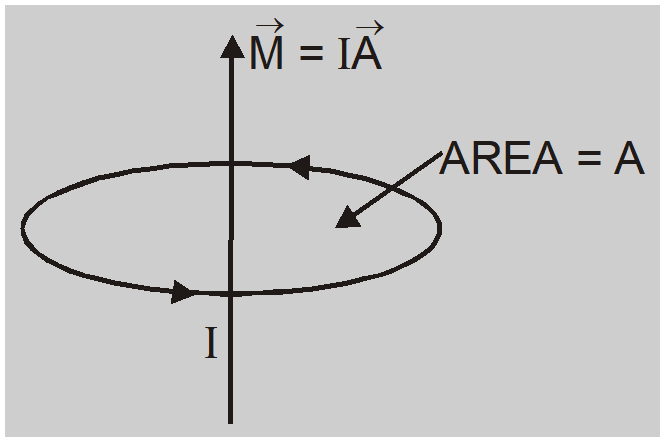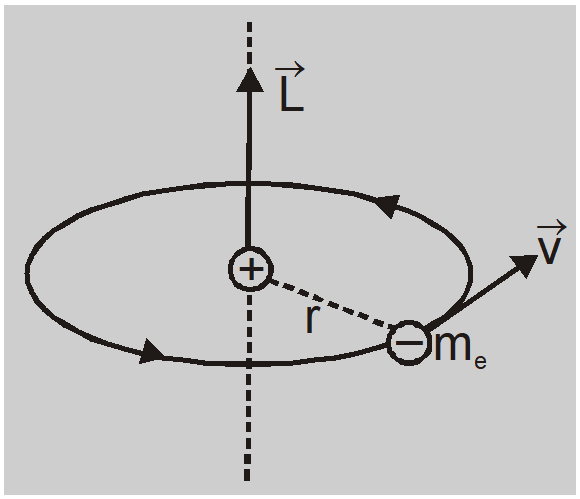Most Affordable JEE | NEET | 8,9,10 Preparation by Kota's Top IITian Doctor Faculties

# Current loop as a Magnetic Dipole - Magnetism | Class 12th Physics Notes`

## Current loop as a Magnetic Dipole

Ampere found that the distribution of magnetic lines of force around a finite current-carrying solenoid is similar to that produced by a bar magnet. This is evident from the fact that a compass needle when moved around these two bodies shows similar deflections. After noting the close resemblance between these two, Ampere demonstrated that a simple current loop behaves like a bar magnet and put forward that all the magnetic phenomena are due to circulating electric current. This is Ampere’s hypothesis.

We consider a circular coil carrying current I. When seen from above current flows in the anti-clockwise direction.1. The magnetic field lines due to each elementary portion of the circular coil are circular near the element and almost straight near the center of the circular coil.2. The magnetic lines of force seem to enter at the lower face of the coil and leave at the upper face.
3. The lower face through which lines of force enter behaves as a south pole and the upper face through which field lines leave behaves as the north pole.
4. A planar loop of any shape behaves as a magnetic dipole.
5. The dipole moment of current loop $(\mathrm{M})=$ ampere turns (nI) $\times$ area of coil (A) or $\mathrm{M}=\mathrm{nIA}$.
6. The unit of dipole moment is ampere meter $^{2}\left( A - m ^{2}\right)$
7. The magnetic dipole moment is a vector with direction from S pole to N pole or along the direction of normal to the planar area.

### Atoms as a Magnetic Dipole

In an atom, electrons revolve around the nucleus. These moving electrons behave as small current loops. So atom possesses a magnetic dipole moment and hence behaves as a magnetic dipole.

The angular momentum of electron due to orbital motion $L = m _{ e } vr$

The equivalent current due to orbital motion $I =-\frac{ e }{ T }=-\frac{ ev }{2 \pi r }$–ve sign shows direction of current is opposite to direction of motion of electron.

Magnetic dipole moment $M=I A=-\frac{e v}{2 \pi r} \cdot \pi r^{2}=-\frac{e v r}{2}$

Using $L=m_{e}$ vr we have $\quad M=-\frac{e}{2 m_{e}} L$

In vector form $\overrightarrow{ M }=-\frac{ e }{2 m _{ e }} \overrightarrow{ L }$

The direction of magnetic dipole moment vector is opposite to angular momentum vector.

According to Bohr's theory $L=\frac{n h}{2 \pi} \quad n=0, \quad 1, \quad 2 \ldots \ldots$

So $M=\left(\frac{e}{2 m_{e}}\right) \frac{n h}{2 \pi}=n\left(\frac{e h}{4 \pi m_{e}}\right)=n \mu_{B}$

Where $\mu_{\mathrm{B}}=\frac{\mathrm{eh}}{4 \pi \mathrm{m}_{e}}$

$=\frac{\left(1.6 \times 10^{-19} \mathrm{C}\right)\left(6.62 \times 10^{-34} \mathrm{Js}\right)}{4 \times 3.14 \times\left(9.1 \times 10^{-31} \mathrm{~kg}\right)}$

$=9.27 \times 10^{-24} \mathrm{Am}^{2}$

is called Bohr Magneton. This is natural unit of magnetic moment.

Biot Savart's Law

Click here for the Video tutorials of Magnetic Effect of Current Class 12
About eSaral At eSaral we are offering a complete platform for IIT-JEE & NEET preparation. The main mission behind eSaral is to provide education to each and every student in India by eliminating the Geographic and Economic factors, as a nation’s progress and development depends on the availability of quality education to each and every one. With the blend of education & technology, eSaral team made the learning personalized & adaptive for everyone.